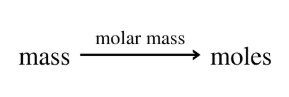# Problem: What is the amount, in moles, of each of the following substances?12.0 g Ar

###### FREE Expert Solution
• Determine the molar mass of Ar then setup a dimensional analysis to find number of moles• According to the periodic table, Ar has a molar mass of 39.95 g/mol

89% (280 ratings)###### Problem Details

What is the amount, in moles, of each of the following substances?

12.0 g Ar